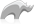##### [ integer ]

Type

The integer data type represents positive or negative whole numbers or zero. Integers are stored as 64-bit signed values.

Integers are usually created as literals. Positive integer literals are simple numbers such as 1, 34, 5018. Negative integer literals are simple numbers preceded by a unary negative symbol: -35, -123.

Integers can be manipulated using a number of mathematical symbols. For example, (1 + 1), (5 - 3), (2 * 3), (10 / 5), or (9 % 10). An expression with an integer on either side of the mathematical symbol will always return an integer value. In particular, (10 / 3) will return the integer 3 rather than the decimal 3.333333.

Integers can be used as storage for bit values using the [Integer->Bit...] methods. See the descriptions of each individual method for more information.

Strings or decimals can be cast to integers using the [Integer] method. For example, [Integer('3')] returns the integer 3 and [Integer('7.333333')] returns the integer 7.

• Syntax
• Methods
• Traits
``````integer
integer('String Value')
integer(Decimal Value)``````
###### Has methods:
• `integer->oncompare(p0::integer)`
• `integer->hash()`
• `integer->+(p0::integer)`
• `integer->+(s::string)`
• `integer->+()::integer`
• `integer->-(p0::integer)`
• `integer->-()`
• `integer->*(p0::integer)`
• `integer->/(p0::integer)`
• `integer->hosttonet16()`
• `integer->hosttonet32()`
• `integer->nettohost16()`
• `integer->nettohost32()`
• `integer->bitset(p0::integer)`
• `integer->bittest(p0::integer)`
• `integer->bitflip(p0::integer)`
• `integer->bitclear(p0::integer)`
• `integer->bitor(p0::integer)`
• `integer->bitand(p0::integer)`
• `integer->bitxor(p0::integer)`
• `integer->bitnot()`
• `integer->bitshiftleft(p0::integer)`
• `integer->bitshiftright(p0::integer)`
• `integer->bytes()`
• `integer->abs()`
• `integer->div(p0::integer)`
• `integer->asString(p0::string, p1::string, p2::string)`
• `integer->asString(-hexaDecimal::boolean = ?, -padding::integer = ?, -padChar::string = ?, -padRight::boolean = ?, -groupchar::string = ?)`
• `integer->dereferencepointer(p0::integer)`
• `integer->asDecimal()`
###### Has traits:
• `any`
• `trait_asString`
• `trait_scalar`
• `trait_serializable`
• Beginner

### To cast a decimal value to an integer:

Use the [Integer] method.

Code

``integer(10.123)``

Result

`10`

### To cast a string as an integer:

Use the [Integer] method.

Code

``integer("10.123")``

Result

`10`Please note that periodically LassoSoft will go through the notes and may incorporate information from them into the documentation. Any submission here gives LassoSoft a non-exclusive license and will be made available in various formats to the Lasso community.